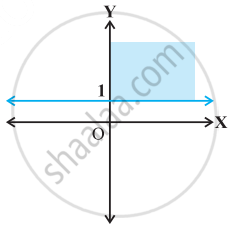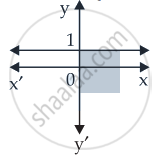# Solution set of x ≥ 0 and y ≤ 1 is - Mathematics

MCQ
True or False

Solution set of x ≥ 0 and y ≤ 1 is• True

• False

#### Solution

Solution set of x ≥ 0 and y ≤ 1  isConcept: Solution of System of Linear Inequalities in Two Variables
Is there an error in this question or solution?

#### APPEARS IN

NCERT Mathematics Exemplar Class 11
Chapter 6 Linear Inequalities
Exercise | Q 31.(xiv) | Page 112

Share